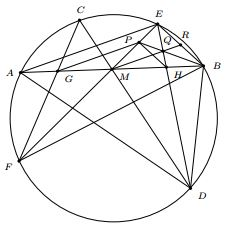# Another synthetic proof of the butterfly theorem using the midline in triangle

### Tran Quang Hung Forum Geometricorum, Volume 16 (2016) 345�346

Let $M\;$ be the midpoint of a chord $AB\;$ of a circle. Through $M\;$ two other chords $CD\;$ and $EF\;$ are drawn. If $C\;$ and $F\;$ are on opposite sides of $AB,\;$ and $CF,\;$ $DE\;$ intersect $AB\;$ at $G\;$ and $H\;$ respectively, then $M\;$ is also the midpoint of $GH.$Let $P\;$ be the point on segment $ME\;$ such that $GP\parallel AE.\;$ $PB\;$ intersects $EH\;$ at $Q.\;$ We have

$\angle PGB = \angle EAB = \angle EFB = \angle PFB.$

This shows that quadrilateral $FGPB\;$ is cyclic. We get

$\angle QBM = \angle PBG = \angle PFG = \angle EFC = \angle EDC = \angle QDM.$

Therefore, quadrilateral $DMQB\;$ is also cyclic. From this,

$\angle QMB = \angle QDB = \angle EDB = \angle EAB,$

and $MQ\parallel AE.\;$ Since $M\;$ is the midpoint of $AB,\;$ by the midline theorem, $MQ\;$ passes through the midpoint $R\;$ of $EB.\;$ By Ceva's theorem for $\Delta MEB\;$ and Thales's theorem for $\Delta MEA,\;$ we get $\displaystyle\frac{MH}{MB} = \frac{MP}{ME} = \frac{MG}{MA}.\;$ Since $MA = MB,\;$ we also have $MG = MH.$### Butterfly Theorem and Variants